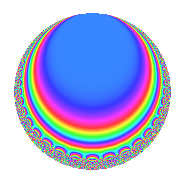Properties

 Label 128.5.d.cLevel 128 Weight 5 Character orbit 128.d Analytic conductor 13.231 Analytic rank 0 Dimension 4 CM No Inner twists 4

Related objects

Newspace parameters

 Level: $$N$$ = $$128 = 2^{7}$$ Weight: $$k$$ = $$5$$ Character orbit: $$[\chi]$$ = 128.d (of order $$2$$ and degree $$1$$)

Newform invariants

 Self dual: No Analytic conductor: $$13.2313552747$$ Analytic rank: $$0$$ Dimension: $$4$$ Coefficient field: $$\Q(i, \sqrt{6})$$ Coefficient ring: $$\Z[a_1, \ldots, a_{5}]$$ Coefficient ring index: $$2^{11}$$ Sato-Tate group: $\mathrm{SU}(2)[C_{2}]$

$q$-expansion

Coefficients of the $$q$$-expansion are expressed in terms of a basis $$1,\beta_1,\beta_2,\beta_3$$ for the coefficient ring described below. We also show the integral $$q$$-expansion of the trace form.

 $$f(q)$$ $$=$$ $$q$$ $$-\beta_{2} q^{3}$$ $$-\beta_{1} q^{5}$$ $$+ \beta_{3} q^{7}$$ $$+ 15 q^{9}$$ $$+O(q^{10})$$ $$q$$ $$-\beta_{2} q^{3}$$ $$-\beta_{1} q^{5}$$ $$+ \beta_{3} q^{7}$$ $$+ 15 q^{9}$$ $$+ 11 \beta_{2} q^{11}$$ $$-27 \beta_{1} q^{13}$$ $$+ \beta_{3} q^{15}$$ $$-162 q^{17}$$ $$-45 \beta_{2} q^{19}$$ $$-96 \beta_{1} q^{21}$$ $$-9 \beta_{3} q^{23}$$ $$+ 561 q^{25}$$ $$+ 66 \beta_{2} q^{27}$$ $$-163 \beta_{1} q^{29}$$ $$-8 \beta_{3} q^{31}$$ $$-1056 q^{33}$$ $$+ 64 \beta_{2} q^{35}$$ $$-189 \beta_{1} q^{37}$$ $$+ 27 \beta_{3} q^{39}$$ $$+ 1890 q^{41}$$ $$-297 \beta_{2} q^{43}$$ $$-15 \beta_{1} q^{45}$$ $$+ 18 \beta_{3} q^{47}$$ $$-3743 q^{49}$$ $$+ 162 \beta_{2} q^{51}$$ $$+ 247 \beta_{1} q^{53}$$ $$-11 \beta_{3} q^{55}$$ $$+ 4320 q^{57}$$ $$+ 231 \beta_{2} q^{59}$$ $$+ 297 \beta_{1} q^{61}$$ $$+ 15 \beta_{3} q^{63}$$ $$-1728 q^{65}$$ $$+ 171 \beta_{2} q^{67}$$ $$+ 864 \beta_{1} q^{69}$$ $$-99 \beta_{3} q^{71}$$ $$+ 2750 q^{73}$$ $$-561 \beta_{2} q^{75}$$ $$+ 1056 \beta_{1} q^{77}$$ $$-102 \beta_{3} q^{79}$$ $$-7551 q^{81}$$ $$-953 \beta_{2} q^{83}$$ $$+ 162 \beta_{1} q^{85}$$ $$+ 163 \beta_{3} q^{87}$$ $$+ 2430 q^{89}$$ $$+ 1728 \beta_{2} q^{91}$$ $$+ 768 \beta_{1} q^{93}$$ $$+ 45 \beta_{3} q^{95}$$ $$+ 7454 q^{97}$$ $$+ 165 \beta_{2} q^{99}$$ $$+O(q^{100})$$ $$\operatorname{Tr}(f)(q)$$ $$=$$ $$4q$$ $$\mathstrut +\mathstrut 60q^{9}$$ $$\mathstrut +\mathstrut O(q^{10})$$ $$4q$$ $$\mathstrut +\mathstrut 60q^{9}$$ $$\mathstrut -\mathstrut 648q^{17}$$ $$\mathstrut +\mathstrut 2244q^{25}$$ $$\mathstrut -\mathstrut 4224q^{33}$$ $$\mathstrut +\mathstrut 7560q^{41}$$ $$\mathstrut -\mathstrut 14972q^{49}$$ $$\mathstrut +\mathstrut 17280q^{57}$$ $$\mathstrut -\mathstrut 6912q^{65}$$ $$\mathstrut +\mathstrut 11000q^{73}$$ $$\mathstrut -\mathstrut 30204q^{81}$$ $$\mathstrut +\mathstrut 9720q^{89}$$ $$\mathstrut +\mathstrut 29816q^{97}$$ $$\mathstrut +\mathstrut O(q^{100})$$

Basis of coefficient ring in terms of a root $$\nu$$ of $$x^{4}\mathstrut +\mathstrut$$ $$9$$:

 $$\beta_{0}$$ $$=$$ $$1$$ $$\beta_{1}$$ $$=$$ $$8 \nu^{2}$$$$/3$$ $$\beta_{2}$$ $$=$$ $$($$$$-4 \nu^{3} + 12 \nu$$$$)/3$$ $$\beta_{3}$$ $$=$$ $$($$$$32 \nu^{3} + 96 \nu$$$$)/3$$
 $$1$$ $$=$$ $$\beta_0$$ $$\nu$$ $$=$$ $$($$$$\beta_{3}\mathstrut +\mathstrut$$ $$8$$ $$\beta_{2}$$$$)/64$$ $$\nu^{2}$$ $$=$$ $$3$$ $$\beta_{1}$$$$/8$$ $$\nu^{3}$$ $$=$$ $$($$$$3$$ $$\beta_{3}\mathstrut -\mathstrut$$ $$24$$ $$\beta_{2}$$$$)/64$$

Character Values

We give the values of $$\chi$$ on generators for $$\left(\mathbb{Z}/128\mathbb{Z}\right)^\times$$.

 $$n$$ $$5$$ $$127$$ $$\chi(n)$$ $$-1$$ $$-1$$

Embeddings

For each embedding $$\iota_m$$ of the coefficient field, the values $$\iota_m(a_n)$$ are shown below.

For more information on an embedded modular form you can click on its label.

Label $$\iota_m(\nu)$$ $$a_{2}$$ $$a_{3}$$ $$a_{4}$$ $$a_{5}$$ $$a_{6}$$ $$a_{7}$$ $$a_{8}$$ $$a_{9}$$ $$a_{10}$$
63.1
 1.22474 + 1.22474i 1.22474 − 1.22474i −1.22474 − 1.22474i −1.22474 + 1.22474i
0 −9.79796 0 8.00000i 0 78.3837i 0 15.0000 0
63.2 0 −9.79796 0 8.00000i 0 78.3837i 0 15.0000 0
63.3 0 9.79796 0 8.00000i 0 78.3837i 0 15.0000 0
63.4 0 9.79796 0 8.00000i 0 78.3837i 0 15.0000 0
 $$n$$: e.g. 2-40 or 990-1000 Significant digits: Format: Complex embeddings Normalized embeddings Satake parameters Satake angles

Inner twists

Char. orbit Parity Mult. Self Twist Proved
1.a Even 1 trivial yes
4.b Odd 1 yes
8.b Even 1 yes
8.d Odd 1 yes

Hecke kernels

This newform can be constructed as the kernel of the linear operator $$T_{3}^{2}$$ $$\mathstrut -\mathstrut 96$$ acting on $$S_{5}^{\mathrm{new}}(128, [\chi])$$.